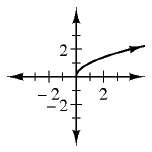### Home > CC3MN > Chapter 6 Unit 10 > Lesson INT1: 6.2.1 > Problem6-54

6-54.

1. Make a table for the rule $y = \sqrt { x }$ that includes $x$‑values from $−1$ to $10$. What happened?

What happens when you substitute a negative answer for $x$?
Does this make sense?

The graph has no value for $x < 0$.

$\begin{array} {c|c} \quad x \quad & \quad y \quad \\ \hline -1 & \\ \hline 0 & 0 \\ \hline 1 & 1 \\ \hline 2 & 1.41 \\ \hline 3 & 1.73 \\ \hline 4 & 2 \\ \hline 5 & 2.24 \\ \hline 6 & 2.45 \\ \hline 7 & 2.65 \\ \hline 8 & 2.83 \\ \hline 9 & 3 \\ \hline 10 & 3.16 \\ \end{array}$

2. Graph the rule on graph paper.3. What kind of growth does the rule show? How do you know?

Do the y-values increase by a constant amount?

4. Is this relationship a function? How do you know?

Is there one output for every input?